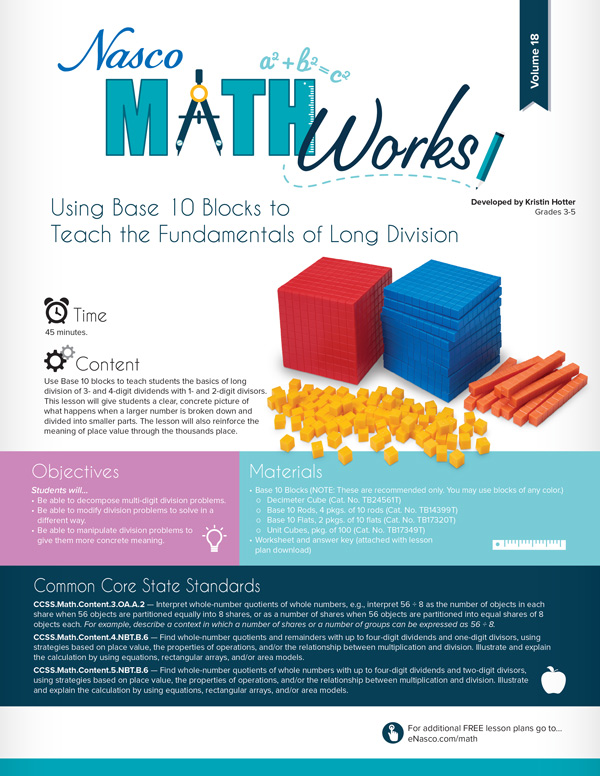# Don’t Let Math Be Confusing!Math can be a confusing subject. Even those who love numbers may get hung up on technicalities. Nasco has the perfect solution for when your students get stuck – Free PDF MathWorks Lesson Plans!  Teachers just like you have created many of our lesson plans. They have discovered an easier way to grasp math concepts and have incorporated them into lessons for all grade levels.

Coordinating a Shape’s Area and Perimeter – Students will construct various shapes on geoboards given specific coordinates, calculate both the area and the perimeter of each polygon, and create their own shapes given specific parameters.

Calculating Wages…How Much Will You Make? – Students will model, write, and solve two-step linear equations using a variety of methods, compare and order rational numbers (positive and negative integers, fractions, decimals) in real-life situations, and use the basic operations on rational numbers to solve problems in real-life situations (e.g. describe the effect of multiplying a whole number by a fraction or a decimal less than 1).

Using Base 10 Blocks to Teach the Fundamentals of Long Division – Students will be able to decompose multi-digit division problems, modify division problems to solve in a different way, and manipulate division problems to give them more concrete meaning.

Graphing Your Story – Students will use simple graphs to practice math skills while reading.

Keva Planks – STEM Lesson Plans – Challenges students to build structures within specific parameters that hold an unlimited number of possible responses.

Dividing Whole Numbers by Unit Fractions –  create, construct, and sketch problems that involve dividing whole numbers by unit fractions.

Pasta Triangles – A Triangle Inequality Activity – Through the use of pasta sticks, students will discover the triangle inequality principle.

Solving Algebraic Equations – Students will be able to solve a variety of linear equations using a balance scale model, explain the process of solving a linear equation, and build concrete models to represent abstract algebraic equations.Rekenrek – Word Problems (Vol. 2) – Students will be able to find the answer to word problems with answers between 0 and 20, design their own real-world word problems with answers between 0 and 20, and utilize an algebraic equation when solving each word problem.

Using 10 Frames for Algebraic Equations Up to 20 – Students will be able to apply basic algebraic principles to addition problems, create fact families with the given digits, and manipulate ten frames to solve addition problems with missing addends.

Nasco has these and many more lesson plans available for free download at our MathWorks website. New lesson plans come out every month!